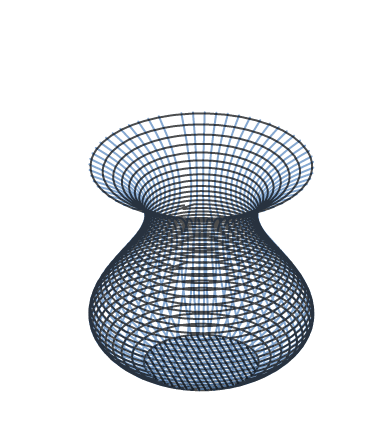# Vase Maker Makes a Vase

Calculus Level 3A vase maker decides to construct a vase whose contour is a cubic polynomial according to the following specifications:

$\bullet$ The vase is 4 feet tall.
$\bullet$ The vase is 4 feet in diameter at its widest (which occurs 1 foot from the base).
$\bullet$ The vase is 2 feet in diameter at its narrowest (which occurs 1 foot from the top).
$\bullet$ The vase has a flat circular bottom.

Rounded to 3 decimal places, what is the volume of the vase in cubic feet?

×